Do you like the article?
Share it with others -

Use new possibilities of MetaTrader 5

# Controlling the Slope of Balance Curve During Work of an Expert Advisor

21 September 2010, 11:11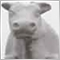5
6 934

### Introduction

This article describes one of approaches, which allows improving performance of Expert Advisors through creation of a feedback. In this case, the feedback will be based on measuring the slope of balance curve. Control of the slope is performed automatically by regulating work volume. An Expert Advisor can trade in the following modes: with a cut volume, with work amount of lots (according to initially adjusted one) and with an intermediate volume. The mode of working is switched automatically.

Different regulating characteristics are used in the feedback chain: stepped, stepped with hysteresis, linear. It allows adjusting the system of controlling the slope of balance curve to the characteristics of a certain system.

The main idea is to automate the process of making decisions for a trader while monitoring own trading system. It's reasonable to cut risks during unfavorable periods of its working. At returning to the normal mode of working risks can be restored back to initial level.

Of course, this system is not a panacea, and it won't turn a losing Expert Advisor to a profitable one. In some way, this is an addition to the MM (money management) of Expert Advisor that keeps it from getting considerable losses at an account.

The article includes a library, which allows embedding this function to code of any Expert Advisor.

### Principle of Operation

Let's take a look into the principle of operation of the system, which controls the slope of balance curve. Assume that we a have a trading Expert Advisor. Its hypothetic curve of balance looks as following:Figure 1. Principle of operation of the system that controls the slope of balance curve

Initial curve of balance for the Expert Advisor that uses constant volume of trade operations is shown above. Closed trades are shown with the red points. Let's connect those points with a curve line, which represents the change of balance of the Expert Advisor during trading (thick black line).

Now we're going to continuously track the angle of slope of this line to the time axis (shown with thin blue lines). Or to be more precise, before opening each trade by a signal, we'll calculate the slope angle by two previously closed trades (or by two trades, for the description to be simpler). If the angle of slope becomes less than the specified value then our controlling system starts working; it decreases the volume according to the calculated value of the angle and the specified regulating function.

In such a manner, if the trade gets into an unsuccessful period, the volume decreases from Vmax. to Vmin. within the Т3...Т5 period of trading. After the Т5 point trading is performed with a minimal specified volume - in the mode of rejection of trade volume. Once the profitability of the Expert Advisor is restored and the angle of slope of the balance curve rises above the specified value, the volume starts increasing. This happens within the Т8...Т10 interval. After the Т10 point, volume of trade operations restores to the initial state Vmax.

The curve of balance formed as a result of such regulation is shown in the lower part of the fig. 1. You can see that the initial drawdown from B1 to B2 has decreased and became from B1 to B2*. You can also observe that the profit slightly decreased within the period of restoring maximum volume Т8...Т10 - this is the reverse of the medal.

Green color highlights the part of the balance curve when trading was performed with minimal specified volume. Yellow color represents the parts of transition from maximum to minimum volume and back. Several variants of transition are possible here:

• stepped - volume changes in discrete steps from maximum to minimum volume and back;
• linear - volume is changed linearly depending in the angle of slope of the balance curve within the regulated interval;
• stepped with hysteresis - transition from maximum to minimum volume and back is performed at difference values of the slope angle;

Let's illustrate it in pictures: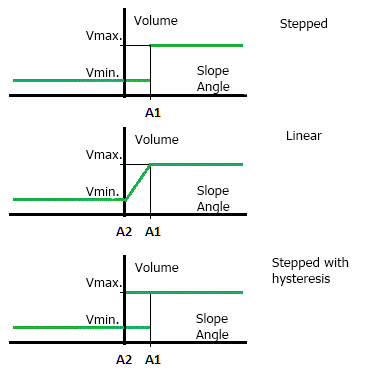Figure 2. Types of regulating characteristics

Regulating characteristics affect the rates of the controlling system - the delay of enabling/disabling, the process of transition from maximum to minimum volume and back. It's recommended to choose a characteristic on experimental basis when reaching the best results of testing.

Thus, we enhance the trading system with the feedback based on the slope angle of the balance curve. Note that such regulation of volume is suitable only for those systems, which don't have the volume as a part of trading system itself. For example, if the Martingale principle is used, you cannot use this system directly without changes in the initial Expert Advisor.

In addition, we need to draw our attention to the following important points:

• the effectiveness of managing the slope of the balance line directly depends on the ratio of work volume in normal mode of operation to the volume in the mode of volume rejection. The greater this ratio is, the more effective the management is. That's why the initial work volume should be considerably greater than the minimum possible one.
• the average period of alteration of rises and falls of the balance of Expert Advisor should be considerably bigger than the time of reaction of the control system. Otherwise, the system won't manage to regulate the slope of the balance curve. The more the ratio of average period to the reaction time is, the more effective the system is. This requirement concerns almost every system of automatic regulation.

### Implementation in MQL5 Using Object-Oriented Programming

Let's write a library that realizes the approach described above. To do it, let's use the new feature of MQL5 - object-oriented approach. This approach allows to easily develop and expand our library in future without rewriting big parts of the code from a scratch.

Since the multi-currency testing is implemented in the new MetaTrader 5 platform, we need a class, which encapsulates in itself the entire working with any work symbol. It allows using this library in multi-currency Expert Advisors. This class doesn't concern the controlling system directly, it's auxiliary. So, this class will be used for operations with the work symbol.

```//---------------------------------------------------------------------
//  Operations with work symbol:
//---------------------------------------------------------------------
{
private:

private:
double  min_trade_volume_step;                 // minimum change of volume
double  max_total_volume;                      // maximum change of volume
double  symbol_point;                          // size of one point
double  symbol_tick_size;                      // minimum change of price
int     symbol_digits;                        // number of digits after decimal point

protected:

public:
void    RefreshSymbolInfo( );                  // refresh market information about the work symbol
void    SetTradeSymbol( string _symbol );      // set/change work symbol
string  GetTradeSymbol( );                     // get work symbol
double  GetMaxTotalLots( );                    // get maximum cumulative volume
double  GetPoints( double _delta );            // get change of price in points

public:
double  NormalizeLots( double _requied_lot );  // get normalized trade volume
double  NormalizePrice( double _org_price );   // get normalized price with consideration of step of change of quote

public:
};```

Structure of the class is very simple. Purpose is getting, storing and processing the current market information by a specified symbol. Main methods are TradeSymbol::RefreshSymbolInfo, TradeSymbol::NormalizeLots, TradeSymbol::NormalizePrice. Let's consider them one by one.

The TradeSymbol::RefreshSymbolInfo method is intended for refreshing the market information by the work symbol.

```//---------------------------------------------------------------------
//  Refresh market information by work symbol:
//---------------------------------------------------------------------
void
{
//  If a work symbol is not set, don't do anything:
if( GetTradeSymbol( ) == NULL )
{
return;
}

//  Calculate parameters necessary for normalization of volume:

max_total_volume = SymbolInfoDouble( GetTradeSymbol( ), SYMBOL_VOLUME_LIMIT );

symbol_point = SymbolInfoDouble( GetTradeSymbol( ), SYMBOL_POINT );
symbol_digits = ( int )SymbolInfoInteger( GetTradeSymbol( ), SYMBOL_DIGITS );
}```

Pay attention to one important point that is used in several methods. Since the current realization of MQL5 doesn't allow using a constructor with parameters, you must call the following method for primary setting of work symbols:

`void    SetTradeSymbol( string _symbol );      // set/change work symbol`

The TradeSymbol::NormalizeLots method is used for getting a correct and normalized volume. We know that the size of a position cannot be less than the minimum possible value allowed by broker. Minimal step of change of a position is also determined by broker, and it can differ. This method returns the closest value of volume from the bottom.

It also checks if the volume of supposed position exceeds the maximum value allowed by broker.

```//---------------------------------------------------------------------
//---------------------------------------------------------------------
//  - input necessary volume;
//  - output is normalized volume;
//---------------------------------------------------------------------
double
{
double   lots, koeff;
int      nmbr;

//  If a work symbol is not set, don't do anything:
if( GetTradeSymbol( ) == NULL )
{
return( 0.0 );
}

{
nmbr = ( int )MathLog10( koeff );
}
else
{
nmbr = 2;
}
lots = MathFloor( _requied_lots * koeff ) / koeff;

//  Lower limit of volume:
{
}

//  Upper limit of volume:
{
}

lots = NormalizeDouble( lots, nmbr );
return( lots );
}```

The TradeSymbol::NormalizePrice method is used for getting correct and normalized price. Since the number of significant digits after the decimal point (accuracy of price) must be determined for a given symbol, we need to truncate the price. In addition to it, some symbols (for example, futures) have a minimum step of price change greater than one point. That's why we need to make the values of price be multiple of minimum discrecity.

```//---------------------------------------------------------------------
//  Normalization of price with consideration of step of price change:
//---------------------------------------------------------------------
double
{
//  Minimal step of quote change in points:
double  min_price_step = NormalizeDouble( symbol_tick_size / symbol_point, 0 );

double  norm_price = NormalizeDouble( NormalizeDouble(( NormalizeDouble( _org_price / symbol_point, 0 )) / min_price_step, 0 ) * min_price_step * symbol_point, symbol_digits );
return( norm_price );
}```

The necessary unnormalized price is inputted to the function. And it returns the normalized price, which is closest to the necessary one.

The purpose of the other methods is clearly described in comments; it doesn't require any further description.

### Class TBalanceHistory

This class, is intended for operating with the history of balance of an account, that is clear for its name. It is also a base class for several classes described below. The main purpose of this class is the access to the trade history of an Expert Advisor. In addition, you can filter the history by work symbol, by "magic number", by date of start of monitoring the Expert Advisor or by all three elements simultaneously.

```//---------------------------------------------------------------------
//  Operations with balance history:
//---------------------------------------------------------------------
class TBalanceHistory
{
private:
long      current_magic;            // value of "magic number" when accessing the history of deals ( 0 - any number )
long      current_type;             // type of deals ( -1 - all )
int       current_limit_history;   // limit of depth of history ( 0 - all history )
datetime   monitoring_begin_date;   // date of start of monitoring history of deals

protected:

protected:
//  "Raw" arrays:
double    org_datetime_array[ ];                                                                                                                                                      // date/time of trade
double    org_result_array[ ];                                                                                                                                                                // result of trade

//  Arrays with data grouped by time:
double    group_datetime_array[ ];                                                                                                                                            // date/time of trade
double    group_result_array[ ];                                                                                                                                                      // result of trade

double    last_result_array[ ];     // array for storing results of last trades ( points on the Y axis )
double    last_datetime_array[ ];   // array for storing time of last trades ( points on the X axis )

private:
void      SortMasterSlaveArray( double& _m[ ], double& _s[ ] );  // synchronous ascending sorting of two arrays

public:
void      SetTradeSymbol( string _symbol );                      // set/change work symbol
string    GetTradeSymbol( );                                    // get work symbol
void      RefreshSymbolInfo( );                                 // refresh market information by work symbol
void      SetMonitoringBeginDate( datetime _dt );                // set date of start of monitoring
datetime  GetMonitoringBeginDate( );                            // get date of start of monitoring
void      SetFiltrParams( long _magic, long _type = -1, int _limit = 0 );// set parameters of filtration of deals

public:
// Get results of last trades:

public:
void      TBalanceHistory( );       // constructor
void      ~TBalanceHistory( );      // destructor
};```

The settings of filtration when reading the results of last trades and history are set using the TBalanceHistory::SetFiltrParams method. It has the following input parameters:

• _magic           - "magic number" of trades that should be read from the history. If the zero value is specified then trades with any "magic number" will be read.
• _limit             - limits the depth of analyzed history of trades. If it's equal to zero, all the available history is analyzed.

On default, the following values are set when the object of the TBalanceHistory class is created: _magic = 0, _type = -1, _limit = 0.

Main method of this class is TBalanceHistory::GetTradeResultsArray. It is intended for filling class member arrays last_result_array and last_datetime_array with the results of last trades. The method has the following input parameters:

• _max_trades - maximum number of trades which should be read from the history and be written to the output arrays. Since we need at least two points to calculate the angle of slope, this value should be no less than two. If this value is equal to zero, the entire available history of trades is analyzed. Practically, the number of points necessary for calculation of slope of the balance curve is specified here.
```//---------------------------------------------------------------------
//---------------------------------------------------------------------
//  - returns the number of actually read trades but not more than specified;
//---------------------------------------------------------------------
int
{
int       index, limit, count;
long      deal_type, deal_magic, deal_entry;
datetime   deal_close_time, current_time;
ulong     deal_ticket;                        // ticket of deal
string    symbol, deal_symbol;

//  Number of trades should be no less than two:
{
return( 0 );
}

//  If a work symbol is not specified, don't do anything:
if( symbol == NULL )
{
return( 0 );
}

//  Request the history of deals and orders from the specified time to the current moment:
if( HistorySelect( monitoring_begin_date, TimeCurrent( )) != true )
{
return( 0 );
}

count = HistoryDealsTotal( );

//  If there are less trades in the history than it is necessary, then exit:
{
return( 0 );
}

//  If there are more trades in the history than it is necessary, then limit them:
if( current_limit_history > 0 && count > current_limit_history )
{
limit = count - current_limit_history;
}
else
{
limit = 0;
}

//  If needed, adjust dimension of "raw" arrays by the specified number of trades:
if(( ArraySize( org_datetime_array )) != ( count - limit ))
{
ArrayResize( org_datetime_array, count - limit );
ArrayResize( org_result_array, count - limit );
}

//  Fill the "raw" array with trades from history base:
for( index = count - 1; index >= limit; index-- )
{
deal_ticket = HistoryDealGetTicket( index );

//  If those are not closed deals, don't go further:
deal_entry = HistoryDealGetInteger( deal_ticket, DEAL_ENTRY );
if( deal_entry != DEAL_ENTRY_OUT )
{
continue;
}

//  Check "magic number" of deal if necessary:
deal_magic = HistoryDealGetInteger( deal_ticket, DEAL_MAGIC );
if( current_magic != 0 && deal_magic != current_magic )
{
continue;
}

//  Check symbol of deal:
deal_symbol = HistoryDealGetString( deal_ticket, DEAL_SYMBOL );
if( symbol != deal_symbol )
{
continue;
}

//  Check type of deal if necessary:
deal_type = HistoryDealGetInteger( deal_ticket, DEAL_TYPE );
if( current_type != -1 && deal_type != current_type )
{
continue;
}
else if( current_type == -1 && ( deal_type != DEAL_TYPE_BUY && deal_type != DEAL_TYPE_SELL ))
{
continue;
}

//  Check time of closing of deal:
deal_close_time = ( datetime )HistoryDealGetInteger( deal_ticket, DEAL_TIME );
if( deal_close_time < monitoring_begin_date )
{
continue;
}

org_datetime_array[ real_trades ] = deal_close_time / 60;
org_result_array[ real_trades ] = HistoryDealGetDouble( deal_ticket, DEAL_PROFIT ) / HistoryDealGetDouble( deal_ticket, DEAL_VOLUME );
}

//  if there are less trades than necessary, return:
{
return( 0 );
}

//  Sort the "raw" array by date/time of closing the order:
SortMasterSlaveArray( org_datetime_array, org_result_array );

// If necessary, adjust dimension of group arrays for the specified number of points:
if(( ArraySize( group_datetime_array )) != count )
{
ArrayResize( group_datetime_array, count );
ArrayResize( group_result_array, count );
}
ArrayInitialize( group_datetime_array, 0.0 );
ArrayInitialize( group_result_array, 0.0 );

//  Fill the output array with grouped data ( group by the identity of date/time of position closing ):
for( index = 0; index < count; index++ )
{
deal_close_time = ( datetime )org_datetime_array[ index ];

//  Now check if the same time already exists in the output array:
current_time = ( datetime )group_datetime_array[ real_trades ];
if( current_time > 0 && MathAbs( current_time - deal_close_time ) > 0.0 )
{
real_trades++;                      // move the pointer to the next element
}
else
{
}
}
real_trades++;                          // now this is the number of unique elements

//  If there are less trades than necessary, exit:
{
return( 0 );
}

if( ArraySize( last_result_array ) != _max_trades )
{
}

//  Write the accumulated data to the output arrays with reversed indexation:
for( index = 0; index < _max_trades; index++ )
{
last_result_array[ _max_trades - 1 - index ] = group_result_array[ index ];
last_datetime_array[ _max_trades - 1 - index ] = group_datetime_array[ index ];
}

//  In the output array replace the results of single trades with the accumulating total:
for( index = 1; index < _max_trades; index++ )
{
last_result_array[ index ] += last_result_array[ index - 1 ];
}

}```

Obligatory checks are performed in the beginning - if a work symbols is specified and if the input parameters are correct.

Then we read the history of deals and orders from the specified date to the current moment. It is performed in the following part of the code:

```//  Request the history of deals and orders from the specified time to the current moment:
if( HistorySelect( monitoring_begin_date, TimeCurrent( )) != true )
{
return( 0 );
}

count = HistoryDealsTotal( );

//  If there are less trades in the history than it is necessary, then exit:
{
return( 0 );
}```

In addition, the total number of deals in the history is checked. If it's less than specified, further actions are meaningless. As soon as the "raw" arrays are prepared, the cycle of filling them with the information from the history of trades is executed. It is done in the following way:

```//  Fill the "raw" array from the base of history of trades:
for( index = count - 1; index >= limit; index-- )
{
deal_ticket = HistoryDealGetTicket( index );

//  If the trades are not closed, don't go further:
deal_entry = HistoryDealGetInteger( deal_ticket, DEAL_ENTRY );
if( deal_entry != DEAL_ENTRY_OUT )
{
continue;
}

//  Check "magic number" of deal if necessary:
deal_magic = HistoryDealGetInteger( deal_ticket, DEAL_MAGIC );
if( _magic != 0 && deal_magic != _magic )
{
continue;
}

//  Check symbols of deal:
deal_symbol = HistoryDealGetString( deal_ticket, DEAL_SYMBOL );
if( symbol != deal_symbol )
{
continue;
}

//  Check type of deal if necessary:
deal_type = HistoryDealGetInteger( deal_ticket, DEAL_TYPE );
if( _type != -1 && deal_type != _type )
{
continue;
}
else if( _type == -1 && ( deal_type != DEAL_TYPE_BUY && deal_type != DEAL_TYPE_SELL ))
{
continue;
}

//  Check time of closing of deal:
deal_close_time = ( datetime )HistoryDealGetInteger( deal_ticket, DEAL_TIME );
if( deal_close_time < monitoring_begin_date )
{
continue;
}

org_datetime_array[ real_trades ] = deal_close_time / 60;
org_result_array[ real_trades ] = HistoryDealGetDouble( deal_ticket, DEAL_PROFIT ) / HistoryDealGetDouble( deal_ticket, DEAL_VOLUME );
}

//  If there are less trades than necessary, exit:
{
return( 0 );
}
```

In the beginning, the ticket of deal from the history is read using the HistoryDealGetTicket function; further reading of deal details is performed using the obtained ticket. Since we are interested only in closed trades (we're going to analyze the balance), the type of deal is checked at first. It is done by calling the HistoryDealGetInteger function with the DEAL_ENTRY parameter. If the function returns DEAL_ENTRY_OUT, then it's closing of a position.

After that "magic number" of the deal, type of the deal (is the input parameter of method is specified) and symbol of the deal are checked. If all the parameters of the deal meet the requirements, then the last parameter is checked - time of closing of the deal. It is done in the following way:

```//  Check the time of closing of deal:
deal_close_time = ( datetime )HistoryDealGetInteger( deal_ticket, DEAL_TIME );
if( deal_close_time < monitoring_begin_date )
{
continue;
}
```

The date/time of the deal is compared with the given date/time of start of monitoring the history. If the date/time of the deal is greater than the given one, then we go to reading our trade to the array - read the result of the trade in points and the time of the trade in minutes (in this case, the time of closing). After that, the counter of read deals real_trades is increased; and the cycle continues.

Once the "raw" arrays are filled with necessary amount of information, we should sort the array where the time of closing of deals is stored. At the same time, we need to keep the correspondence of time of closing in the org_datetime_array array and the results of deals in the org_result_array array. This is done using the specially written method:

TBalanceHistory::SortMasterSlaveArray( double& _master[ ], double& _slave[ ] ). First parameter is _master - the array which is sorted in ascending way. Second parameter is _slave - the array, the elements of which should be moved synchronously with the elements of the first array. The sorting is performed via the "bubble" method.

After all operations described above, we have two arrays with time and results of deals sorted by time. Since only one point on the balance curve (point on the Y axis) can correspond to each moment of time (point on the X axis), we need to group the elements of the array with the same time of closing (if there are). The following part of the code performs this operation:

```//  Fill the output array with grouped data ( group by identity of date/time of closing of position ):
for( index = 0; index < count; index++ )
{
deal_close_time = ( datetime )org_datetime_array[ index ];

//  Now check, if the same time already exists in the output array:
current_time = ( datetime )group_datetime_array[ real_trades ];
if( current_time > 0 && MathAbs( current_time - deal_close_time ) > 0.0 )
{
real_trades++;                      // move the pointer to the next element
}
else
{
}
}
real_trades++;                          // now this is the number of unique elements
```

Practically, all trades with the "same" time of closing are summed here. The results are written to the TBalanceHistory::group_datetime_array (time of closing) and TBalanceHistory::group_result_array (results of trades) arrays. After that we get two sorted arrays with unique elements. The identity of time in this case is considered within a minute. This transformation can be graphically illustrated:Figure 3. Grouping deals with the same time

All deals within a minute (left part of the figure) are grouped in a single one with rounding of time and summing the results (right part of the figure). It allows smoothing the "chattering" of time of closing deals and improving the stability of regulation.

After that you need to make another two transformations of the obtained arrays. Reverse the order of elements to make the earliest deal correspond to the zero element; and replace the results of single trades with the cumulative total, i.e. with the balance. It is done in the following fragment of the code:

```//  Write the accumulated data into output arrays with reversed indexation:
for( index = 0; index < _max_trades; index++ )
{
last_result_array[ _max_trades - 1 - index ] = group_result_array[ index ];
last_datetime_array[ _max_trades - 1 - index ] = group_datetime_array[ index ];
}

//  Replace the results of single trades with the cumulative total in the output array:
for( index = 1; index < _max_trades; index++ )
{
last_result_array[ index ] += last_result_array[ index - 1 ];
}
```

### Class TBalanceSlope

This class is intended for making operations with the balance curve of an account. It is spawned from the TBalanceHistory class; and it inherits all its protected and public data and methods. Let's take a detailed look in its structure:

```//---------------------------------------------------------------------
//  Operations with the balance curve:
//---------------------------------------------------------------------
class TBalanceSlope : public TBalanceHistory
{
private:
double    current_slope;               // current angle of slope of the balance curve
int       slope_count_points;          // number of points ( trades ) for calculation of slope angle

private:
double    LR_koeff_A, LR_koeff_B;      // rates for the equation of the straight-line regression
double    LR_points_array[ ];          // array of point of the straight-line regression

private:
void      CalcLR( double& X[ ], double& Y[ ] );  // calculate the equation of the straight-line regression

public:
void      SetSlopePoints( int _number );        // set the number of points for calculation of angle of slope
double    CalcSlope( );                         // calculate the slope angle

public:
void      TBalanceSlope( );                     // constructor
void      ~TBalanceSlope( );                    // destructor
};```

We will determine the slope angle of the balance curve by the slope angle of the line of linear regression drawn for the specified amount of points (trades) on the balance curve. Thus, first of all, we need to calculate the equation of the straight-line regression of the following form: A*x + B. The following method does this job:

```//---------------------------------------------------------------------
//  Calculate the equation of the straight-line regression:
//---------------------------------------------------------------------
//  input parameters:
//    X[ ] - arras of values of number series on the X axis;
//    Y[ ] - arras of values of number series on the Y axis;
//---------------------------------------------------------------------
void
TBalanceSlope::CalcLR( double& X[ ], double& Y[ ] )
{
double    mo_X = 0, mo_Y = 0, var_0 = 0, var_1 = 0;
int       i;
int       size = ArraySize( X );
double    nmb = ( double )size;

//  If the number of points is less than two, the curve cannot be calculated:
if( size < 2 )
{
return;
}

for( i = 0; i < size; i++ )
{
mo_X += X[ i ];
mo_Y += Y[ i ];
}
mo_X /= nmb;
mo_Y /= nmb;

for( i = 0; i < size; i++ )
{
var_0 += ( X[ i ] - mo_X ) * ( Y[ i ] - mo_Y );
var_1 += ( X[ i ] - mo_X ) * ( X[ i ] - mo_X );
}

//  Value of the A coefficient:
if( var_1 != 0.0 )
{
LR_koeff_A = var_0 / var_1;
}
else
{
LR_koeff_A = 0.0;
}

//  Value of the B coefficient:
LR_koeff_B = mo_Y - LR_koeff_A * mo_X;

//  Fill the array of points that lie on the regression line:
ArrayResize( LR_points_array, size );
for( i = 0; i < size; i++ )
{
LR_points_array[ i ] = LR_koeff_A * X[ i ] + LR_koeff_B;
}
}```

Here we use the method of least squares to calculate the minimum error of position of the regression line relatively to the initial data. The array that stores the Y coordinates, which lie on the calculated line, is also filled. This array is not used for the time being and is meant for further development.

The main method that is used in the given class is TBalanceSlope::CalcSlope. It returns the slope angle of the balance curve, which is calculated by the specified amount of last trades. Here is its realization:

```//---------------------------------------------------------------------
//  Calculate slope angle:
//---------------------------------------------------------------------
double
TBalanceSlope::CalcSlope( )
{
int      nmb = GetTradeResultsArray( slope_count_points );
if( nmb < slope_count_points )
{
return( 0.0 );
}

//  Calculate the regression line by the results of last trades:
CalcLR( last_datetime_array, last_result_array );
current_slope = LR_koeff_A;

return( current_slope );
}```

First of all, the specified amount of last points of the balance curve is analyzed. It is done by calling the method of the base class TBalanceSlope::GetTradeResultsArray. If the amount of read points is not less than specified, the regression line is calculated. It is done using the TBalanceSlope::CalcLR method. Filled at the previous step, the last_result_array and last_datetime_array arrays, which belong to the base class, are used as arguments.

The rest of methods are simple and don't require a detailed description.

### Class TBalanceSlopeControl

It is the base class, which manages the slope of the balance curve by modifying the work volume. It is spawned from the TBalanceSlope class, and it inherits all its public and protected methods and data. The only purpose of this class is to calculate the current work volume depending on the current angle of slope of the balance curve. Let's take a detailed look into it:

```//---------------------------------------------------------------------
//  Managing slope of the balance curve:
//---------------------------------------------------------------------
enum LotsState
{
LOTS_NORMAL = 1,            // mode of trading with normal volume
LOTS_REJECTED = -1,         // mode of trading with lowered volume
LOTS_INTERMEDIATE = 0,      // mode of trading with intermediate volume
};
//---------------------------------------------------------------------
class TBalanceSlopeControl : public TBalanceSlope
{
private:
double    min_slope;          // slope angle that corresponds to the mode of volume rejection
double    max_slope;          // slope angle that corresponds to the mode of normal volume
double    centr_slope;        // slope angle that corresponds to the mode of volume switching without hysteresis

private:
ControlType  control_type;    // type of the regulation function

private:
double    rejected_lots;      // volume in the rejection mode
double    normal_lots;        // volume in the normal mode
double    intermed_lots;      // volume in the intermediate mode

private:
LotsState current_lots_state; // current mode of volume

public:
void      SetControlType( ControlType _control );  // set type of the regulation characteristic
void      SetControlParams( double _min_slope, double _max_slope, double _centr_slope );

public:

protected:
double    CalcIntermediateLots( double _min_lots, double _max_lots, double _slope );

public:
void      TBalanceSlopeControl( );   // constructor
void      ~TBalanceSlopeControl( );  // destructor
};```

Before calculating the current volume, we need to set initial parameters. It is done by calling the following methods:

```  void      SetControlType( ControlType _control );  // set type of the regulation characteristic
```

Input parameter_control - this is the type of the regulation characteristic. It can have the following value:

• STEP_WITH_HYSTERESISH      - stepped with hysteresis regulation characteristic;
• STEP_WITHOUT_HYSTERESIS  - stepped without hysteresis regulation characteristic;
• LINEAR                                   - linear regulation characteristic;
• NON_LINEAR                           - non-linear regulation characteristic (not implemented in this version);

```  void      SetControlParams( double _min_slope, double _max_slope, double _centr_slope );
```

Input parameters are following:

• _min_slope - slope angle of the balance curve that corresponds to trading with minimal volume;
• _max_slope - slope angle of the balance curve that corresponds to trading with maximal volume;
• _centr_slope - slope angle of the balance curve that corresponds to the stepped regulation characteristic without hysteresis;

The volume is calculated using the following method:

```//---------------------------------------------------------------------
//---------------------------------------------------------------------
double
TBalanceSlopeControl::CalcTradeLots( double _min_lots, double _max_lots )
{
//  Try to calculate slope of the balance curve:
double    current_slope = CalcSlope( );

//  If the specified amount of trades is not accumulated yet, trade with minimal volume:
if( GetRealTrades( ) < GetSlopePoints( ))
{
current_lots_state = LOTS_REJECTED;
return( rejected_lots );
}

//  If the regulation function is stepped without hysteresis:
if( control_type == STEP_WITHOUT_HYSTERESIS )
{
if( current_slope < centr_slope )
{
current_lots_state = LOTS_REJECTED;
return( rejected_lots );
}
else
{
current_lots_state = LOTS_NORMAL;
return( normal_lots );
}
}

//  If the slope of linear regression for the balance curve is less than the allowed one:
if( current_slope < min_slope )
{
current_lots_state = LOTS_REJECTED;
return( rejected_lots );
}

//  If the slope of linear regression for the balance curve is greater than specified:
if( current_slope > max_slope )
{
current_lots_state = LOTS_NORMAL;
return( normal_lots );
}

//  The slope of linear regression for the balance curve is within specified borders (intermediate state):
current_lots_state = LOTS_INTERMEDIATE;

//  Calculate the value of intermediate volume:
intermed_lots = CalcIntermediateLots( _min_lots, _max_lots, current_slope );

return( intermed_lots );
}```

Main significant points of implementation of the TBalanceSlopeControl::CalcTradeLots method are following:

• Until the specified minimal amount of trades is accumulated, trade with minimal volume. It's logical, because it's not known, which period (profitable or not) the Expert Advisor is currently in, right after you set it for trading.
• If the regulation function is the one stepped without hysteresis, then to set the angle of switching between the modes of trading via the TBalanceSlopeControl::SetControlParams method you should use only the _centr_slope parameter. The _min_slope and _max_slope parameters are ignored. It is done to perform the correct optimization by this parameter in the MetaTrader 5 strategy tester.

Depending on the calculated angle of slope, trading is performed with minimal, maximal or intermediate volume. Intermediate volume is calculated via the simple method - TBalanceSlopeControl::CalcIntermediateLots. This method is protected and it's used within the class. Its code is shown below:

```//---------------------------------------------------------------------
//  Calculation of intermediate volume:
//---------------------------------------------------------------------
double
TBalanceSlopeControl::CalcIntermediateLots( double _min_lots, double _max_lots, double _slope )
{
double    lots;

//  If the regulation function is stepped with hysteresis:
if( control_type == STEP_WITH_HYSTERESISH )
{
if( current_lots_state == LOTS_REJECTED && _slope > min_slope && _slope < max_slope )
{
lots = _min_lots;
}
else if( current_lots_state == LOTS_NORMAL && _slope > min_slope && _slope < max_slope )
{
lots = _max_lots;
}
}
//  If the regulation function is linear:
else if( control_type == LINEAR )
{
double  a = ( _max_lots - _min_lots ) / ( max_slope - min_slope );
double  b = normal_lots - a * .max_slope;
lots = a * _slope + b;
}
//  If the regulation function is non-linear ( not implemented yet ):
else if( control_type == NON_LINEAR )
{
lots = _min_lots;
}
//  If the regulation function is unknown:
else
{
lots = _min_lots;
}

return( lots );
}```

Other methods of this class don't require any description.

### Example of Embedding the System into an Expert Advisor

Let's consider the process of implementation of the system of controlling the slope of the balance curve in an Exert Advisor step by step.

Step 1 - adding the instruction to connect the developed library to the Expert Advisor:

`#include  <BalanceSlopeControl.mqh>`

Step 2 - adding the external variables for setting parameters of the system of controlling the slope of the balance line to the Expert Advisor:

```//---------------------------------------------------------------------
//  Parameters of the system of controlling the slope of the balance curve;
//---------------------------------------------------------------------
enum SetLogic
{
No = 0,
Yes = 1,
};
//---------------------------------------------------------------------
input SetLogic     UseAutoBalanceControl = No;
//---------------------------------------------------------------------
input ControlType  BalanceControlType = STEP_WITHOUT_HYSTERESIS;
//---------------------------------------------------------------------
//  Amount of last trades for calculation of LR of the balance curve:
//---------------------------------------------------------------------
//  Slope of LR to decrease the volume to minimum:
input double       LRKoeffForRejectLots = -0.030;
//---------------------------------------------------------------------
//  Slope of LR to restore the normal mode of trading:
input double       LRKoeffForRestoreLots = 0.050;
//---------------------------------------------------------------------
//  Slope of LR to work in the intermediate mode:
input double       LRKoeffForIntermedLots = -0.020;
//---------------------------------------------------------------------
//  Decrease the initial volume to the specified value when the LR is inclined down
input double       RejectedLots = 0.10;
//---------------------------------------------------------------------
//  Normal work volume in the mode of MM with fixed volume:
input double       NormalLots = 1.0;```

Step 3 - adding the object of the TBalanceSlopeControl type to the Expert Advisor:

`TBalanceSlopeControl  BalanceControl;`

This declaration can be added at the beginning of the Expert Advisor, before the definitions of functions.

Step 4 - adding the code for initialization of the system of controlling of the balance curve to the OnInit function of the Expert Advisor:

```//  Adjust our system of controlling the slope of the balance curve:
BalanceControl.SetControlType( BalanceControlType );
BalanceControl.SetControlParams( LRKoeffForRejectLots, LRKoeffForRestoreLots, LRKoeffForIntermedLots );
BalanceControl.SetFiltrParams( 0, -1, 0 );
BalanceControl.SetMonitoringBeginDate( 0 );```

Step 5 - adding the call of method for refreshing the current market information to the OnTick function of the Expert Advisor:

```//  Refresh market information:
BalanceControl.RefreshSymbolInfo( );```

The call of this method can be added to the very beginning of the OnTick function or after the check of new bar coming (for Expert Advisors with such check).

Step 6 - adding the code for calculation of current volume before the code where positions are opened:

```  if( UseAutoBalanceControl == Yes )
{
current_lots = BalanceControl.CalcTradeLots( RejectedLots, NormalLots );
}
else
{
current_lots = NormalLots;
}```

If a Money Management system is used in the Expert Advisor, then instead the NormalLots you should write the TBalanceSlopeControl::CalcTradeLots method - the current volume calculated by the MM system of the Expert Advisor.

Test Expert Advisor BSCS-TestExpert.mq5 with the in-built system described above is attached to this article. Principle of its operation is based on intersection of levels of the CCI indicator. This Expert Advisor is developed for testing and is not suitable for working on real accounts. We're going to test it at the H4 timeframe (2008.07.01 - 2010.09.01) of EURUSD.

Let's analyze the result of working of this EA. The chart of change of balance with the system of controlling the slope disabled is shown below. To see it, set the No value for the UseAutoBalanceControl external parameter.Figure 4. Initial chart of change of balance

Now set the UseAutoBalanceControl external parameter to Yes and test the Expert Advisor. You will get the chart with the enabled system of controlling the slope of balance.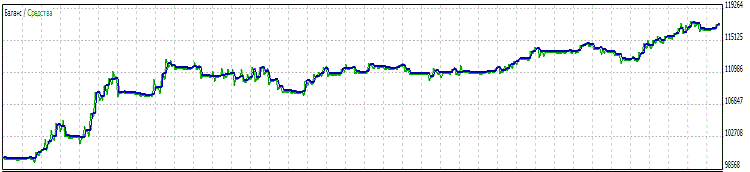Figure 5. Chart of change of balance with the system of controlling enabled

You can see that most of the periods at the upper chart (fig.4) look as they are cut, and they have a flat form at the lower chart (fig.5). This is the result of working of our system. You can compare the main parameters of working of the Expert Advisor:

Parameter
UseAutoBalanceControl = No  UseAutoBalanceControl = Yes
Total net profit: 18 378.0017 261.73
Profit factor: 1.471.81
Recovery factor: 2.66 3.74
Expected payoff:  117.81110.65
Absolute drawdown of balance:1 310.50131.05
Absolute drawdown of equity: 1 390.50514.85
Maximal drawdown of balance: 5 569.50 (5.04%) 3 762.15 (3.35%)
Maximal drawdown of equity:6 899.50 (6.19%)
4 609.60 (4.08%)

Best parameters among the compared ones are highlighted with the green color. Profit and expected payoff have slightly decreased; this is the other side of regulation, which appears as a result of lags of switching between the states of work volume. All in all, there is an improvement of rates of working of the Expert Advisor. Especially, improvement of drawdown and profit factor.

### Conclusion

I see several ways of improving this system:
• Using virtual trading when the Expert Advisor enters an unfavorable period of working. Then the normal work volume won't matter anymore. It will allow decreasing the drawdown.
• Using more complex algorithms for determining the current state of working of the Expert Advisor (profitable or not). For example, we can try applying a neuron net for such analysis. Additional investigation is needed in this case, of course.

Thus, we have considered the principle and the result of working of the system, which allows improving quality characteristics of an Expert Advisor. Joint operation with the system of money management, in some cases, allows increasing the profitability without increasing the risk.

I remind you once again: no auxiliary system can make a profitable Expert Advisor from a losing one.

Translated from Russian by MetaQuotes Software Corp.
Original article: https://www.mql5.com/ru/articles/145

Attached files |
Last comments | Go to discussion (5)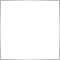| 21 Sep 2010 at 12:52

The article stated: "This method returns the closest value of volume from the bottom."

Method TradeSymbol:: NormalizeLots () in the case

"// Lower limit of volume:
{
}
"

returns the closest value of volume from the top.

It looks like a bug in either article, or in the method.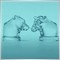| 23 Sep 2010 at 09:08
That's a truly great and inspiring article, definitely one of the best so far on MQL5.com. Thanks to the Author for sharing such valuable knowledge with the rest of the community.| 23 Sep 2010 at 10:41
Enigma71fx:
That's a truly great and inspiring article, definitely one of the best so far on MQL5.com. Thanks to the Author for sharing such valuable knowledge with the rest of the community.
Thanks!| 8 Oct 2010 at 21:48

Could someone help clarify the last comment in the article that stated a possible improvement would be, "Using virtual trading when the Expert Advisor enters an unfavorable period of working. Then the normal work volume won't matter anymore. It will allow decreasing the drawdown." Does this mean that by using virtual trading one could "rebound" from a drawdown more quickly since the diminished volume of trading used during negative periods will no longer affect the balance curve?

Thanks| 10 Oct 2010 at 21:13
ALtrend:

Could someone help clarify the last comment in the article that stated a possible improvement would be, "Using virtual trading when the Expert Advisor enters an unfavorable period of working. Then the normal work volume won't matter anymore. It will allow decreasing the drawdown." Does this mean that by using virtual trading one could "rebound" from a drawdown more quickly since the diminished volume of trading used during negative periods will no longer affect the balance curve?

Thanks

Not more quickly, but with less losses.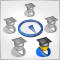Adaptive Trading Systems and Their Use in the MetaTrader 5 Client Terminal

This article suggests a variant of an adaptive system that consists of many strategies, each of which performs its own "virtual" trade operations. Real trading is performed in accordance with the signals of a most profitable strategy at the moment. Thanks to using of the object-oriented approach, classes for working with data and trade classes of the Standard library, the architecture of the system appeared to be simple and scalable; now you can easily create and analyze the adaptive systems that include hundreds of trade strategies.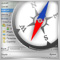Several Ways of Finding a Trend in MQL5

Any trader would give a lot for opportunity to accurately detect a trend at any given time. Perhaps, this is the Holy Grail that everyone is looking for. In this article we will consider several ways to detect a trend. To be more precise - how to program several classical ways to detect a trend by means of MQL5.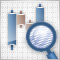Analyzing Candlestick Patterns

Construction of Japanese candlestick chart and analysis of candlestick patterns constitute an amazing area of technical analysis. The advantage of candlesticks is that they represent data in such a manner that you can track the dynamics inside the data. In this article we analyze candlestick types, classification of candlestick patterns and present an indicator that can determine candlestick patterns.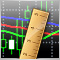Evaluation of Trade Systems - the Effectiveness of Entering, Exiting and Trades in General

There are a lot of measures that allow determining the effectiveness and profitability of a trade system. However, traders are always ready to put any system to a new crash test. The article tells how the statistics based on measures of effectiveness can be used for the MetaTrader 5 platform. It includes the class for transformation of the interpretation of statistics by deals to the one that doesn't contradict the description given in the "Statistika dlya traderov" ("Statistics for Traders") book by S.V. Bulashev. It also includes an example of custom function for optimization.